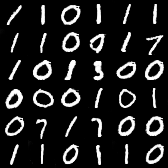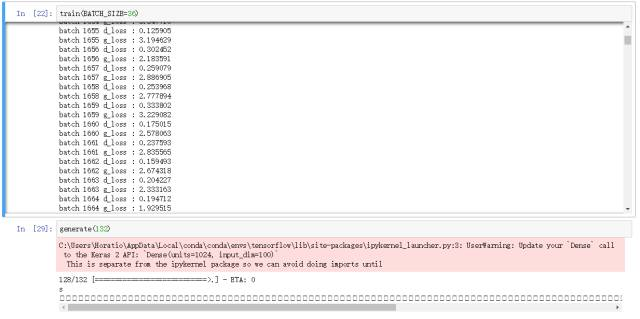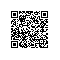# GAN完整理论推导、证明与实现（附代码）

• 第一部分描述 GAN 的直观概念

• 第二部分描述概念与优化的形式化表达

• 第三部分将对 GAN 进行详细的理论推导与分析

• 最后我们将实现前面的理论分析。

GitHub项目地址：

https://github.com/jiqizhixin/ML-Tutorial-Experiment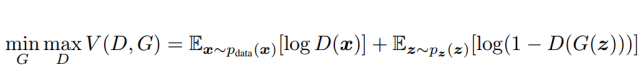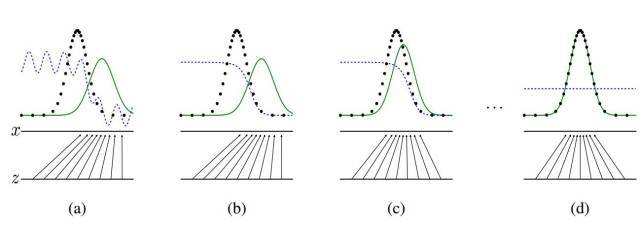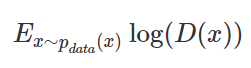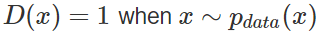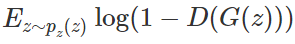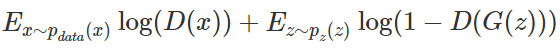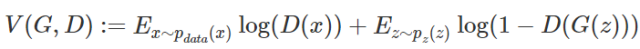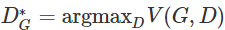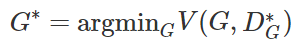KL 散度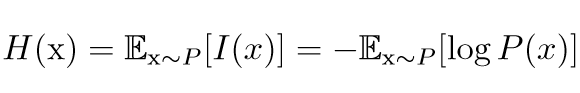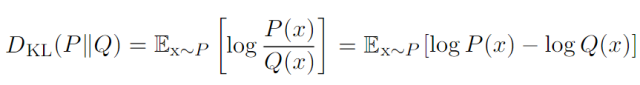KL 散度有很多有用的性质，最重要的是它是非负的。KL 散度为 0 当且仅当 P 和 Q 在离散型变量的情况下是相同的分布，或者在连续型变量的情况下是 『几乎处处』 相同的。因为 KL 散度是非负的并且衡量的是两个分布之间的差异，它经常 被用作分布之间的某种距离。然而，它并不是真的距离因为它不是对称的：对于某些 P 和 Q，D_KL(P||Q) 不等于 D_KL(Q||P)。这种非对称性意味着选择 D_KL(P||Q) 还是 D_KL(Q||P) 影响很大。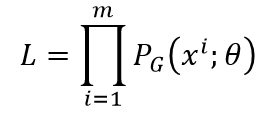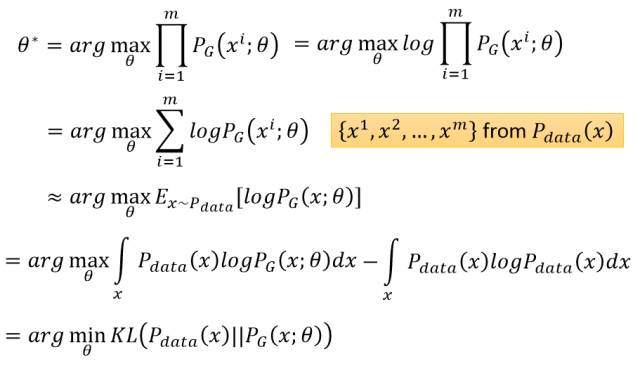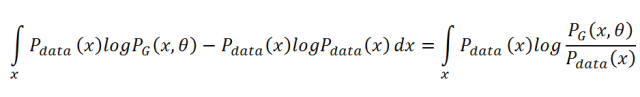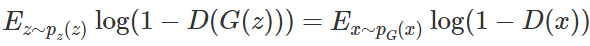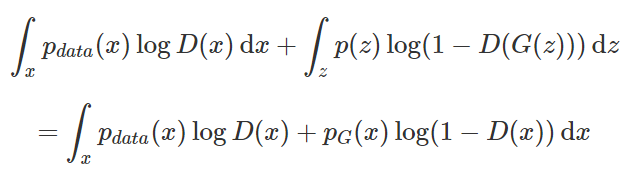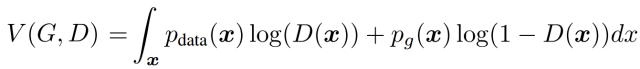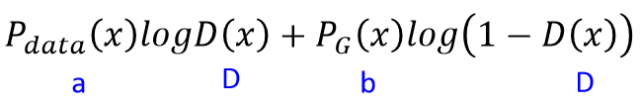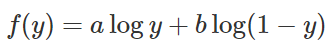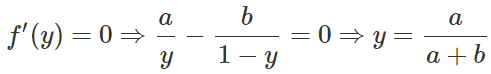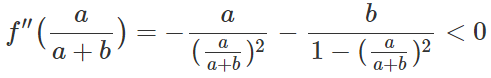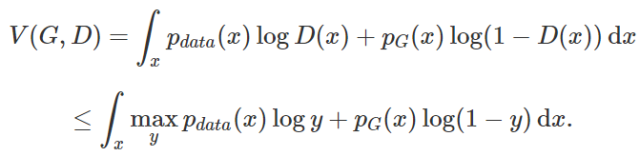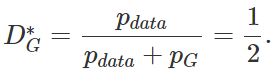「当且仅当 P_G=P_data，训练标准 C(G)=maxV(G,D) 的全局最小点可以达到。」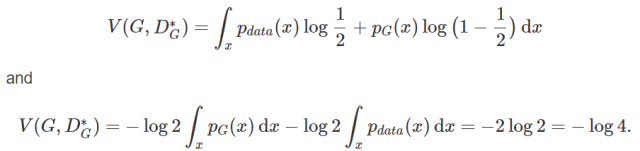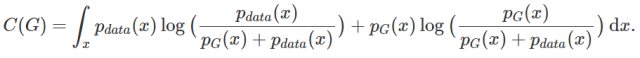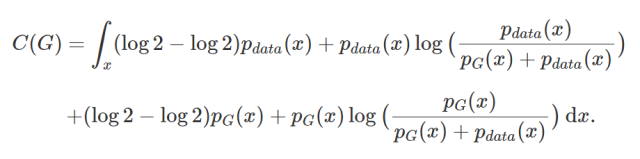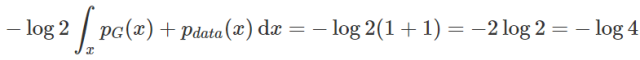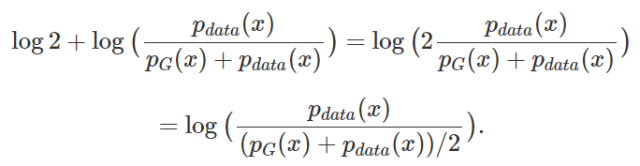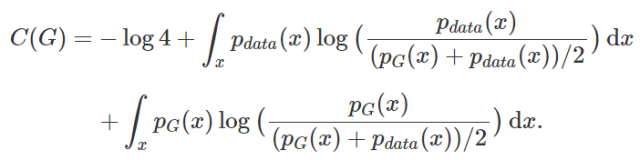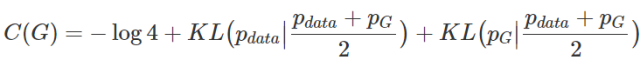KL 散度是非负的，所以我们马上就能看出来 -log4 为 C(G) 的全局最小值。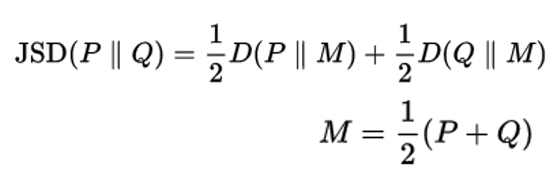假设存在两个分布 P 和 Q，且这两个分布的平均分布 M=(P+Q)/2，那么这两个分布之间的 JS 散度为 P 与 M 之间的 KL 散度加上 Q 与 M 之间的 KL 散度再除以 2。

JS 散度的取值为 0 到 log2。若两个分布完全没有交集，那么 JS 散度取最大值 log2；若两个分布完全一样，那么 JS 散度取最小值 0。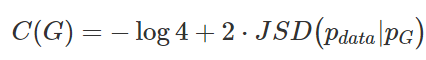• 参数优化过程：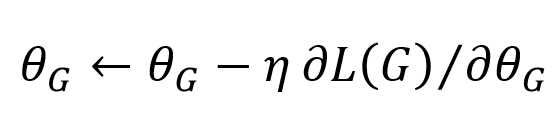•  给定 G_0，最大化 V(G_0,D) 以求得 D_0*，即 max[JSD(P_data(x)||P_G0(x)]；

• 固定 D_0*，计算θ_G1 ← θ_G0 −η(dV(G,D_0*) /dθ_G) 以求得更新后的 G_1；

• 固定 G_1，最大化 V(G_1,D_0*) 以求得 D_1*，即 max[JSD(P_data(x)||P_G1(x)]；

• 固定 D_1*，计算θ_G2 ← θ_G1 −η(dV(G,D_0*) /dθ_G) 以求得更新后的 G_2；

• 。。。

• 实际训练过程：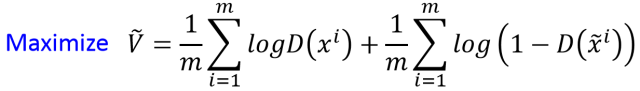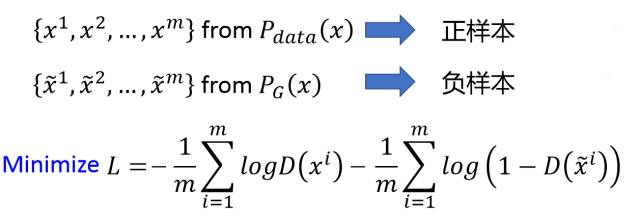• 从真实数据分布 P_data 抽取 m 个样本

• 从先验分布 P_prior(z) 抽取 m 个噪声样本

• 将噪声样本投入 G 而生成数据，通过最大化 V 的近似而更新判别器参数θ_d，即极大化，且判别器参数的更新迭代式为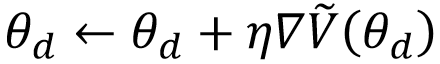• 从先验分布 P_prior(z) 中抽取另外 m 个噪声样本 {z^1,...,z^m}

• 通过极小化 V^tilde 而更新生成器参数θ_g，即极大化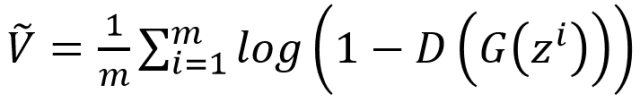，且生成器参数的更新迭代式为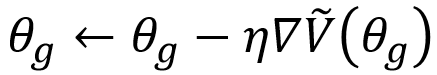GitHub 实现地址：

https://github.com/jiqizhixin/ML-Tutorial-Experiment

1. def generator_model():

2.     #下面搭建生成器的架构，首先导入序贯模型（sequential），即多个网络层的线性堆叠

3.     model = Sequential()

4.     #添加一个全连接层，输入为100维向量，输出为1024维

5.     model.add(Dense(input_dim=100, output_dim=1024))

6.     #添加一个激活函数tanh

7.     model.add(Activation('tanh'))

8.     #添加一个全连接层，输出为128×7×7维度

9.     model.add(Dense(128*7*7))

10.     #添加一个批量归一化层，该层在每个batch上将前一层的激活值重新规范化，即使得其输出数据的均值接近0，其标准差接近1

11.     model.add(BatchNormalization())

12.     model.add(Activation('tanh'))

13.     #Reshape层用来将输入shape转换为特定的shape，将含有128*7*7个元素的向量转化为7×7×128张量

14.     model.add(Reshape((7, 7, 128), input_shape=(128*7*7,)))

15.     #2维上采样层，即将数据的行和列分别重复2次

16.     model.add(UpSampling2D(size=(2, 2)))

17.     #添加一个2维卷积层，卷积核大小为5×5，激活函数为tanh，共64个卷积核，并采用padding以保持图像尺寸不变

18.     model.add(Conv2D(64, (5, 5), padding='same'))

19.     model.add(Activation('tanh'))

20.     model.add(UpSampling2D(size=(2, 2)))

21.     #卷积核设为1即输出图像的维度

22.     model.add(Conv2D(1, (5, 5), padding='same'))

23.     model.add(Activation('tanh'))

24.     return model

1. def generator_containing_discriminator(g, d):

2.     #将前面定义的生成器架构和判别器架构组拼接成一个大的神经网络，用于判别生成的图片

3.     model = Sequential()

4.     #先添加生成器架构，再令d不可训练，即固定d

5.     #因此在给定d的情况下训练生成器，即通过将生成的结果投入到判别器进行辨别而优化生成器

6.     model.add(g)

7.     d.trainable = False

8.     model.add(d)

9.     return model

1. def discriminator_model():

2.     #下面搭建判别器架构，同样采用序贯模型

3.     model = Sequential()

4.       #添加2维卷积层，卷积核大小为5×5，激活函数为tanh，输入shape在‘channels_first’模式下为（samples,channels，rows，cols）

5.     #在‘channels_last’模式下为（samples,rows,cols,channels），输出为64维

6.     model.add(

7.             Conv2D(64, (5, 5),

8.             padding='same',

9.             input_shape=(28, 28, 1))

10.             )

11.     model.add(Activation('tanh'))

12.     #为空域信号施加最大值池化，pool_size取（2，2）代表使图片在两个维度上均变为原长的一半

13.     model.add(MaxPooling2D(pool_size=(2, 2)))

14.     model.add(Conv2D(128, (5, 5)))

15.     model.add(Activation('tanh'))

16.     model.add(MaxPooling2D(pool_size=(2, 2)))

17.     #Flatten层把多维输入一维化，常用在从卷积层到全连接层的过渡

18.     model.add(Flatten())

19.     model.add(Dense(1024))

20.     model.add(Activation('tanh'))

21.     #一个结点进行二值分类，并采用sigmoid函数的输出作为概念

22.     model.add(Dense(1))

23.     model.add(Activation('sigmoid'))

24.     return model

• 加载 MNIST 数据

• 将数据分割为训练与测试集，并赋值给变量

• 设置训练模型的超参数

• 编译模型的训练过程

• 在每一次迭代内，抽取生成图像与真实图像，并打上标注

• 随后将数据投入到判别模型中，并进行训练与计算损失

• 固定判别模型，训练生成模型并计算损失，结束这一次迭代

1. def train(BATCH_SIZE):

2.     # 国内好像不能直接导入数据集，我们试了几次都不行，后来将数据集下载到本地'~/.keras/datasets/'，也就是当前目录（我的是用户文件夹下）下的.keras文件夹中。

3.     #下载的地址为：https://s3.amazonaws.com/img-datasets/mnist.npz

4.     (X_train, y_train), (X_test, y_test) = mnist.load_data()

5.     #iamge_data_format选择"channels_last"或"channels_first"，该选项指定了Keras将要使用的维度顺序。

6.     #"channels_first"假定2D数据的维度顺序为(channels, rows, cols)，3D数据的维度顺序为(channels, conv_dim1, conv_dim2, conv_dim3)

7. 

8.     #转换字段类型，并将数据导入变量中

9.     X_train = (X_train.astype(np.float32) - 127.5)/127.5

10.     X_train = X_train[:, :, :, None]

11.     X_test = X_test[:, :, :, None]



1.     # X_train = X_train.reshape((X_train.shape, 1) + X_train.shape[1:])

2.     #将定义好的模型架构赋值给特定的变量

3.     d = discriminator_model()

4.     g = generator_model()

5.     d_on_g = generator_containing_discriminator(g, d)

6.     #定义生成器模型判别器模型更新所使用的优化算法及超参数

7.     d_optim = SGD(lr=0.001, momentum=0.9, nesterov=True)

8.     g_optim = SGD(lr=0.001, momentum=0.9, nesterov=True)

9.     #编译三个神经网络并设置损失函数和优化算法，其中损失函数都是用的是二元分类交叉熵函数。编译是用来配置模型学习过程的

10.     g.compile(loss='binary_crossentropy', optimizer="SGD")

11.     d_on_g.compile(loss='binary_crossentropy', optimizer=g_optim)

12.     #前一个架构在固定判别器的情况下训练了生成器，所以在训练判别器之前先要设定其为可训练。

13.     d.trainable = True

14.     d.compile(loss='binary_crossentropy', optimizer=d_optim)

15.     #下面在满足epoch条件下进行训练

16.     for epoch in range(30):

17.         print("Epoch is", epoch)

18.         #计算一个epoch所需要的迭代数量，即训练样本数除批量大小数的值取整；其中shape就是读取矩阵第一维度的长度

19.         print("Number of batches", int(X_train.shape/BATCH_SIZE))

20.         #在一个epoch内进行迭代训练

21.         for index in range(int(X_train.shape/BATCH_SIZE)):

22.             #随机生成的噪声服从均匀分布，且采样下界为-1、采样上界为1，输出BATCH_SIZE×100个样本；即抽取一个批量的随机样本

23.             noise = np.random.uniform(-1, 1, size=(BATCH_SIZE, 100))

24.             #抽取一个批量的真实图片

25.             image_batch = X_train[index*BATCH_SIZE:(index+1)*BATCH_SIZE]

26.             #生成的图片使用生成器对随机噪声进行推断；verbose为日志显示，0为不在标准输出流输出日志信息，1为输出进度条记录

27.             generated_images = g.predict(noise, verbose=0)

28.             #每经过100次迭代输出一张生成的图片

29.             if index % 100 == 0:

30.                 image = combine_images(generated_images)

31.                 image = image*127.5+127.5

32.                 Image.fromarray(image.astype(np.uint8)).save(

33.                     "./GAN/"+str(epoch)+"_"+str(index)+".png")

34.             #将真实的图片和生成的图片以多维数组的形式拼接在一起，真实图片在上，生成图片在下

35.             X = np.concatenate((image_batch, generated_images))

36.             #生成图片真假标签，即一个包含两倍批量大小的列表；前一个批量大小都是1，代表真实图片，后一个批量大小都是0，代表伪造图片

37.             y =  * BATCH_SIZE +  * BATCH_SIZE

38.             #判别器的损失；在一个batch的数据上进行一次参数更新

39.             d_loss = d.train_on_batch(X, y)

40.             print("batch %d d_loss : %f" % (index, d_loss))

41.             #随机生成的噪声服从均匀分布

42.             noise = np.random.uniform(-1, 1, (BATCH_SIZE, 100))

43.             #固定判别器

44.             d.trainable = False

45.             #计算生成器损失；在一个batch的数据上进行一次参数更新

46.             g_loss = d_on_g.train_on_batch(noise,  * BATCH_SIZE)

47.             #令判别器可训练

48.             d.trainable = True

49.             print("batch %d g_loss : %f" % (index, g_loss))

50.             #每100次迭代保存一次生成器和判别器的权重

51.             if index % 100 == 9:

52.                 g.save_weights('generator', True)

53.                 d.save_weights('discriminator', True)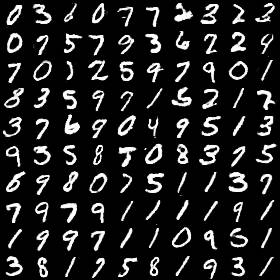1. #batch_size=32

2. batch 2000 d_loss : 0.000318

3. batch 2000 g_loss : 7.618911

1. #batch_size=36

2. batch 2000 g_loss : 1.663281

3. batch 2000 d_loss : 0.483616